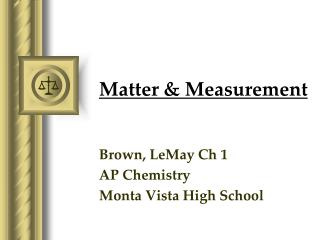# Matter & Measurement - PowerPoint PPT PresentationDownload PresentationMatter & Measurement

Matter & Measurement
Download Presentation## Matter & Measurement

- - - - - - - - - - - - - - - - - - - - - - - - - - - E N D - - - - - - - - - - - - - - - - - - - - - - - - - - -
##### Presentation Transcript

1. Matter & Measurement Brown, LeMay Ch 1 AP Chemistry Monta Vista High School

2. 1.2 & 1.3: The Basics • States of matter: solid, liquid, gas, plasma, BEC • Elements: substances that cannot be decomposed into simpler substances • Compounds: substances composed of two or more elements • Law of constant composition, or law of definite proportions: the relative masses of elements are fixed in a given chemical substance. • Mixtures: combinations of two or more substances • Techniques for separating mixtures: filtration, distillation, chromatography • Properties: • Physical vs. chemical: Did the sample (really) change? • Intensive vs. extensive: Does the measurement depend on quantity of sample?

3. Classification of Matter

4. Classification of Matter

5. Classification of Matter

6. Classification of Matter

7. Classification of Matter

8. Classification of Matter

9. Classification of Matter

10. Classification of Matter

11. Classification of Matter

12. Classification of Matter

13. 1.4: S.I. (the Metric System)

14. kilo k 103 deci d 10-1 centi c 10-2 milli m 10-3 micro m 10-6 nano n 10-9 pico p 10-12

15. 1.5: Uncertainty • Precision: how closely individual measurements agree with one another; the “fineness” of a measurement • Accuracy: how closely individual measurements agree with the “true” value • Significant figures: for any measurement, all the digits that are “certain” plus one “uncertain” digit; an indication of precision

16. Determining Significant Figures • Any nonzero digit is significant. 457 cm 29 cm • Any zero between nonzero digits is significant. 1005 kg 807 kg • Any zero at the “beginning” of a number is not significant; it’s a place holder. 0.0026 Å 0.41 Å = 3 SF = 2 SF = 4 SF = 3 SF = 2 SF = 2 SF Anders Ångström(1814 – 1874)

17. = 4 SF = 1 SF • Any zero at the “end” of a number and after the decimal point is significant. 0.05000 K 3000 K • If a number ends with a decimal point, assume that all digits are significant. 7000. J 20. J • For exact numbers(e.g. 4 beakers) and those used in conversion factors (e.g. 1 inch = 2.54 cm), there is no uncertainty in their measurement. Therefore, IGNORE exact numbers when finalizing your answer with the correct number of significant figures. • For more practice: http://lectureonline.cl.msu.edu/~mmp/applist/sigfig/sig.htm = 4 SF = 2 SF

18. Calculating with Sig Figs • Addition & subtraction: a sum or difference may be no more precise than the least precise measurement. Consider the fewer number of decimal places. Ex: 15.047 g + 4.12 g = ? 15.047 + 4.12 19.167 → 19.17 g Ex: 25,040 mL + 37,200 mL = ? 25040 + 37200 62240 → 62,200 mL or 6.22 x 104 mL

19. Calculating with Sig Figs • Multiplication & division: a product or quotient may be no more significant than the least significant measurement. Consider the fewer number of significant figures. Ex: 3.000 x 4.00 = • 12.0 • (4 SF) x (3 SF) = (3 SF)

20. Logarithms: retain in the mantissa (the “decimal part” of the logarithm) the same number of SF there are in the original value. • log (3.000 x 104) = log (4 SF) → 4 SF in mantissa • log (3 x 104) = log (1 SF) → 1 SF in mantissa • Series of operations: keep all non-significant digits during the intermediate calculations, and round to the correct number of SF only when reporting an answer. Ex: (4.5 + 3.50001) x 2.00 = • AP Exam grading allows for answers to be off by +/- 1 SF without penalty. Example: If the correct answer is 46.2 mL, 46 mL or 46.21 mL are also acceptable. • 4.4771 4.5 (8.00001) x 2.00 = 16.0002 → 16

21. 42.68 g → 42.7 g 17.32 m → 17.3 m 2.7851 cm → 2.79 cm 2.78500 cm → 1.655 cm → 1.66 cm 78.65 mL → 78.6 mL

22. 1.6: Dimensional Analysis • What is the volume (in3) of a 0.500 lb sample of Pb? (d = 11.34 g/mL)

23. Properties and Changes of Matter

24. Types of Properties • Physical Properties… • Can be observed without changing a substance into another substance. • Boiling point, density, mass, volume, etc. • Chemical Properties… • Can only be observed when a substance is changed into another substance. • Flammability, corrosiveness, reactivity with acid, etc.

25. Types of Properties • Intensive Properties… • Are independent of the amount of the substance that is present. • Density, boiling point, color, etc. • Extensive Properties… • Depend upon the amount of the substance present. • Mass, volume, energy, etc.

26. Separation of Mixtures

27. Hand Separation Separate a mixture like this… …using your hand!

28. Filtration In filtration solid substances are separated from liquids and solutions. What remains on the filter paper is called the “precipitate” and what passes through is called the “filtrate.”

29. Separating funnel Shake and let sit… Usually used to separate immiscible liquids, like oil and water mixture.

30. Centrifuge Separates particles of different masses based on centrifugal force. Heavier particles settle at the bottom followed by the lighter particles on top. Place in centrifuge…

31. Distillation Distillation uses differences in the boiling points of substances to separate a homogeneous mixture into its components.

32. Chromatography This technique separates substances on the basis of differences in solubility in a solvent.